# Engineering Mechanics - Equilibrium of a Particle

### Exercise :: Equilibrium of a Particle - General Questions

• Equilibrium of a Particle - General Questions
6.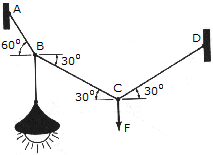Determine the force F needed to hold the 4-kg lamp in the position shown.

 A. F = 39.2 N B. F = 68.0 N C. F = 34.0 N D. F = 19..62 N

Explanation:

No answer description available for this question. Let us discuss.

7.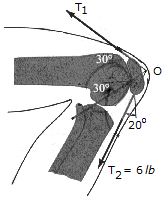The patella P located in the human knee joint is subjected to tendon forces T1 and T2 and a force F exerted on the patella by the femoral articular A. If the directions of these forces are estimated from an X-ray as shown, determine the magnitudes of T1 and F when the tendon force T2 = 6 lb.. The forces are concurrent at point O.

 A. T1 = 1.042 lb, F = 5.91 lb B. T1 = 5.64 lb, F = 5.64 lb C. T1 = 1.203 lb, F = 5.31 lb D. T1 = 4.45 lb, F = 6.82 lb

Explanation:

No answer description available for this question. Let us discuss.

8.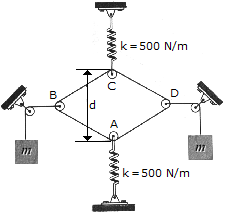A continuous of total length 4 m is wrapped around the small frictionless pulleys at A, B, C, and D. If the stiffness of each spring is k = 500 N/m and each spring is stretched 300 mm, determine the mass m of each block. Neglect the weight of the pulleys and cords. The springs are unstretched when d = 2 m.

 A. m = 153.0 kg B. m = 15.60 kg C. m = 4.75 kg D. m = 30.5 kg

Explanation:

No answer description available for this question. Let us discuss.

9.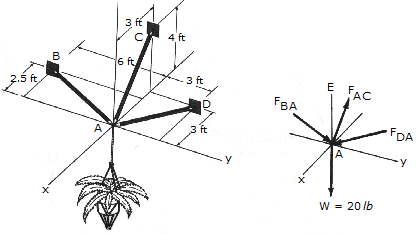Determine the force in each strut and tell whether it is in tension or compression.

 A. Fab = 1.76 lb T, Fac = 5.00 lb T, Fad = 3.53 lb C B. Fab = 11.47 lb T, Fac = 25.0 lb C, Fad = 14.97 lb C C. Fab = 11.47 lb C, Fac = 25.0 lb T, Fad = 14.97 lb C D. Fab = 1.76 lb C, Fac = 5.00 lb T, Fad = 3.53 lb C

Explanation:

No answer description available for this question. Let us discuss.

10.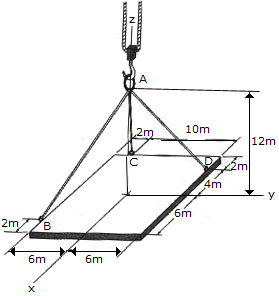The ends of the three cables are attached to ring at A and to the edge of a uniform 150-kg plate. Determine the tension in each of the cables for equilibrium.

 A. Fab = 629 N, Fac = 1030N, Fad = 57.0 N B. Fab = 1116 N, Fac = 1030N, Fad = 429 N C. Fab = 720 N, Fac = 116.1N, Fad = 830 N D. Fab = 858 N, Fac = 0, Fad = 858 N